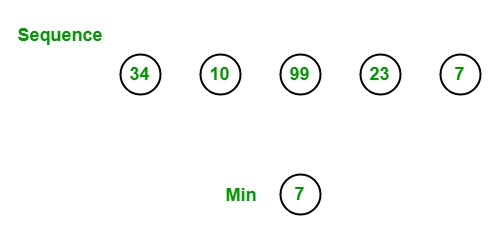# LINQ | How to find minimum value of the given sequence?

In LINQ, you can find the minimum element of the given sequence by using Min() function. This method provides the minimum element of the given set of values. It does not support query syntax in C#, but it supports in VB.NET. It is available in both Enumerable and Queryable classes in C#. It returns null if all the elements present in the collection are null. It returns any type of data type means you can also use Min with the collection of a custom type(does not contain numeric value), but for this, you have to implement the IComparable interface. It can work with nullable, non-nullable decimal, double, floats, int, etc. values.Example 1:

 `// C# program to find minimum ` `// value from the given array ` `using` `System; ` `using` `System.Linq; ` ` `  `class` `GFG { ` ` `  `    ``// Main Method ` `    ``static` `public` `void` `Main() ` `    ``{ ` ` `  `        ``// Data source ` `        ``int``[] sequence = {20, 45, 50, 79, 90, ` `                       ``79, 89, 100, 567, 29}; ` ` `  `        ``// Display the sequence ` `        ``Console.WriteLine(``"The sequence is: "``); ` ` `  `        ``foreach``(``int` `s ``in` `sequence) ` `        ``{ ` `            ``Console.WriteLine(s); ` `        ``} ` ` `  `        ``// Finding the minimum element ` `        ``// from the given sequence ` `        ``// Using Min function ` `        ``int` `result = sequence.Min(); ` `        ``Console.WriteLine(``"Minimum Value: {0}"``, result); ` `    ``} ` `} `

Output:

```The sequence is:
20
45
50
79
90
79
89
100
567
29
Minimum Value: 20
```

Example 2:

 `// C# program to find the Minimum ` `// salary of the employee ` `using` `System; ` `using` `System.Linq; ` `using` `System.Collections.Generic; ` ` `  `// Employee details ` `class` `Employee { ` ` `  `    ``public` `int` `emp_id ` `    ``{ ` `        ``get``; ` `        ``set``; ` `    ``} ` `    ``public` `string` `emp_name ` `    ``{ ` `        ``get``; ` `        ``set``; ` `    ``} ` `    ``public` `string` `emp_gender ` `    ``{ ` `        ``get``; ` `        ``set``; ` `    ``} ` `    ``public` `string` `emp_hire_date ` `    ``{ ` `        ``get``; ` `        ``set``; ` `    ``} ` `    ``public` `int` `emp_salary ` `    ``{ ` `        ``get``; ` `        ``set``; ` `    ``} ` `} ` ` `  `class` `GFG { ` ` `  `    ``// Main method ` `    ``static` `public` `void` `Main() ` `    ``{ ` `        ``List emp = ``new` `List() { ` `            ``new` `Employee() { emp_id = 209, emp_name = ``"Anjita"``, emp_gender = ``"Female"``, emp_hire_date = ``"12/3/2017"``, emp_salary = 20000 }, ` `                ``new` `Employee() { emp_id = 210, emp_name = ``"Soniya"``, emp_gender = ``"Female"``, emp_hire_date = ``"22/4/2018"``, emp_salary = 30000 }, ` `                ``new` `Employee() { emp_id = 211, emp_name = ``"Rohit"``, emp_gender = ``"Male"``, emp_hire_date = ``"3/5/2016"``, emp_salary = 40000 }, ` `                ``new` `Employee() { emp_id = 212, emp_name = ``"Supriya"``, emp_gender = ``"Female"``, emp_hire_date = ``"4/8/2017"``, emp_salary = 40000 }, ` `                ``new` `Employee() { emp_id = 213, emp_name = ``"Anil"``, emp_gender = ``"Male"``, emp_hire_date = ``"12/1/2016"``, emp_salary = 40000 }, ` `                ``new` `Employee() { emp_id = 214, emp_name = ``"Anju"``, emp_gender = ``"Female"``, emp_hire_date = ``"17/6/2015"``, emp_salary = 50000 }, ` `        ``}; ` ` `  `        ``// Find the minimum salary  ` `        ``// of the employee ` `        ``// Using Min () method ` `        ``var` `res = emp.Min(a => a.emp_salary); ` `        ``Console.WriteLine(``"Minimum salary of the employee: {0}"``, res); ` `    ``} ` `} `

Output:

```Minimum salary of the employee: 20000
```

My Personal Notes arrow_drop_upCheck out this Author's contributed articles.

If you like GeeksforGeeks and would like to contribute, you can also write an article using contribute.geeksforgeeks.org or mail your article to contribute@geeksforgeeks.org. See your article appearing on the GeeksforGeeks main page and help other Geeks.

Please Improve this article if you find anything incorrect by clicking on the "Improve Article" button below.

Article Tags :

Be the First to upvote.

Please write to us at contribute@geeksforgeeks.org to report any issue with the above content.